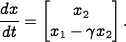Errata: Missing γ in equation (4.9)

The middle expression in equation (4.9) is missing a factor of. The equation should read